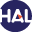# Volume 6 N°1 (2009)

Latest addition : 6 December 2009.

• ### A Discrete Duality Finite Volume method for elliptic problems with corner singularities

6 December 2009,

Full Title: A Discrete Duality Finite Volume method for elliptic problems with corner singularities

Abstract: We focus on the Discrete Duality Finite Volume (DDFV) method whose particularity is to allow the use of unstructured or nonconforming meshes. We deal with the Laplacian problem on nonconvex domains. We show how appropriate refinement conditions on the diamond mesh lead to an optimal order of convergence as for smooth solutions. These theoretical results are illustrated by some numerical applications.

Paper presented by: Fayssal BenkhaldounVoir sur HAL : https://hal.archives-ouvertes.fr/hal-00868421

• ### Error estimates for a finite volume method for the Laplace equation in dimension one through discrete Green functions

6 December 2009,

Full Title:

Error estimates for a finite volume method for the Laplace equation in dimension one through discrete Green functions

Abstract: The cell-centered finite volume approximation of the Laplace equation in dimension one is considered. An exact expression of the error between the exact and numerical solutions is derived through the use of continuous and discrete Green functions. This allows to discuss convergence of the method in the L∞ and L2 norms with respect to the choice of the control points in the cells and with respect to the regularity of the data. Well-known second-order convergence results are recovered if those control points are properly chosen and if the data belongs to H 1 . Counterexamples are constructed to show that second-order may be lost if these conditions are not met.

Paper presented by: Frédéric PascalVoir sur HAL : https://hal.archives-ouvertes.fr/cea-00391590

• ### A 2D/3D Discrete Duality Finite Volume Scheme. Application to ECG simulation

6 December 2009,

Full Title: A 2D/3D Discrete Duality Finite Volume Scheme. Application to ECG simulation

Abstract:

This paper deals with finite volume schemes for elliptic problems in- cluding anisotropy and heterogeneity. The scheme analytical and nu- merical properties are analysed. Its application to a problem arising in bio-mathematics is displayed.

Paper presented by: J.M. HerardVoir sur HAL : https://hal.archives-ouvertes.fr/hal-00328251

• ### Various Approaches for Solving Problems in Heat Conduction with Phase Change

6 December 2009,

Full Title: Various Approaches for Solving Problems in Heat Conduction with Phase Change

Abstract:

This paper treats a one dimensional phase-change problem, ’ice melting’, by a vertex-centered finite volume method. Numerical solutions are obtained by using two approaches where the first one is based on the heat conduction equation with the basic grid (improved by introducing a new adaptive mesh technique), latent heat source approach (LHA), while the second uses the equivalent thermodynamic parameters defined by considering the apparent heat capacity method (AHC).

A comparison between the two approaches is presented, furthermore the accuracy and flexibility of the numerical methods are verified by comparing the results with existing analytical solutions. Results indicate that phase-change problems can be handled easily with excellent accuracies by using the AHC method.

Paper presented by: F. Benkhaldoun and J.M. HerardVoir sur HAL : https://hal.archives-ouvertes.fr/hal-01120384

• ### Error analysis of the Penalty-Projection Method for the Time Dependent Stokes Equations

6 December 2009,

Full Title: Error analysis of the Penalty-Projection Method for the Time Dependent Stokes Equations

Abstract:

We address in this paper a fractional-step scheme for the simulation of incompressible flows falling in the class of penalty-projection methods. The velocity prediction is similar to a penalty method prediction step, or, equivalently, differs from the incremental projection method one by the introduction of a penalty term built to enforce the divergence-free constraint.

Then, a projection step based on a pressure Poisson equation is performed, to update the pressure and obtain an (approximately) divergence-free end-of-step velocity. An analysis in the energy norms for the model unsteady Stokes problem shows that this scheme enjoys the time convergence properties of both underlying methods: for low value of the penalty parameter r, the splitting error estimates of the so-called rotational projection scheme are recovered, i.e. convergence as δt2 and δt3/2 for the velocity and the pressure, respectively; for high values of the penalty parameter, we obtain the δt/r behaviour for the velocity error known for the penalty scheme, together with a 1/r behaviour for the pressure error.

Some numerical tests are presented, which substantiate this analysis.

Paper presented by: Jean-Marc HérardVoir sur HAL : https://hal.archives-ouvertes.fr/hal-00482143